# Test: Sequences

## 20 Questions MCQ Test Quantitative Reasoning for GMAT | Test: Sequences

Description
Attempt Test: Sequences | 20 questions in 40 minutes | Mock test for Quant preparation | Free important questions MCQ to study Quantitative Reasoning for GMAT for Quant Exam | Download free PDF with solutions
QUESTION: 1

### The nth term of an increasing sequence S is given by Sn = Sn-1 + Sn-2 for n > 2 and the nth term of a sequence S’ is given by S’n = S’n-1 - S’n-2 for n > 2. If S5 = S’5, what is the average (arithmetic mean) of S2 and S’2? (1) The difference between the fourth term and the second term of sequence S is 14. (2) The sum of the fourth term and the second term of sequence S’ is 14.

Solution:

Steps 1 & 2: Understand Question and Draw Inferences

• Increasing sequence S whose nth term is represented as  Sn = Sn-1 + Sn-2 for n > 2
• For a sequence S’, the nth term of which is represented as S’n = S’n-1 - S’n-2 for n > 2
• S5 = S’5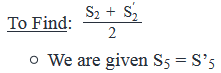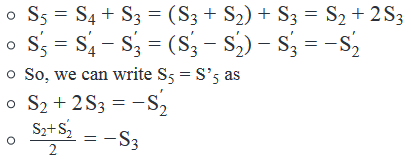Thus we need to find the value of S3 to find the average of S2 andS′2

Step 3: Analyze Statement 1 independently

(1) The difference between the fourth term and the second term of sequence S is 14

• S4−S2=14
• Substituting the expression of S4=S3+S2
• (S3+S2)−S2=14,i.e.S3=14

As we know the value of S3, the statement is sufficient to answer.

Step 4: Analyze Statement 2 independently

(2) The sum of the fourth term and the second term of sequence S’ is 14.

• S′2+S′4=14
• Substituting the expression of S′4=S′3−S′2
• S′2+(S′3−S′2)=14
• S′3=14

Does not tell us anything about the value of S3, the statement is insufficient to answer

Step 5: Analyze Both Statements Together (if needed)

As we have a unique answer from step -3, this step is not required.

QUESTION: 2

### If the sum of the first 30 positive odd integers is k, what is the sum of first 30 non-negative even integers?

Solution:

Given

• 1 + 3 + 5………..30*2 -1 = k.
• Let’s call this sequence O

To Find: 0 + 2+ 4………30 *2 -2 = ?

• Let’s call this sequence E

Approach

1. To express the sum of sequence E in terms of k, we need to express the terms of sequence E in terms of sequence O
1. Now, we see that we can write 0 = 1 – 1
2. Similarly, we can write 2 = 3 -1
3. Continuing the same pattern, we can write 58 = 59 -1
4. Observe that 1, 3…..59 are terms of sequence O. So, using the above process we have captured all the terms of sequence O in sequence E
2. We will use the above logic to represent the sum of sequence E in terms of k

Working Out

1. 0 + 2+ 4……58 = (1-1) + (3-1) +…….(59- 1) = 1+ 3+ 5…….59 – (1 + 1 + 1……….30 times)
2. 0 + 2 + 4 …….+ 58 = k – 30

QUESTION: 3

### 240 students are to be arranged in n rows in the assembly hall of a school. If  the first row is the closest to the stage and each subsequent row has 10 more students than the row ahead of it, what is the value of n? (1) There are 45 students in the 4th row from the stage. (2) The number of students in the nth row is 10 less than 5 times the number of students in the first row.

Solution:

Steps 1 & 2: Understand Question and Draw Inferences

• As the number of students in a row is 10 more than the number of students in the previous row, the number of students in the rows form an arithmetic sequence with common difference, d = 10
• Number of rows = n
• Let the number of students in the first row be a1

and that in the last row be an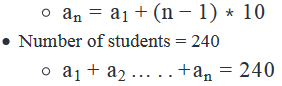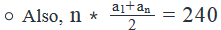(average of the 1st and the nth term is the average of all the terms of the sequence)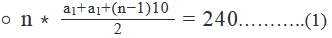To Find: Unique value of n

• Need to find the value of a1  or express a1  in terms of n to find the value of n.

Step 3: Analyze Statement 1 independently

(1) There are 45 students in the 4th row from the stage.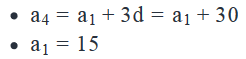Substituting the value of a1 in (1), we have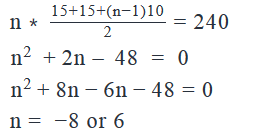As n cannot be negative, n = 6.

Step 4: Analyze Statement 2 independently

(2) The number of students in the nth row is 10 less than 5 times the number of students in the first row.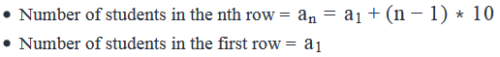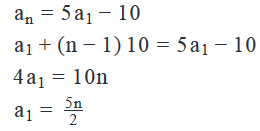Putting the value of in (1), we have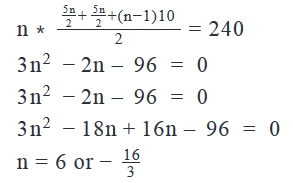As n has to be an integer, n = 6.

Step 5: Analyze Both Statements Together (if needed)

As we have a unique answer from steps 3 and 4, this step is not required.

QUESTION: 4

An increasing sequence P consists of 10 distinct integers. How many integers of the sequence are less than 16?

(1) The difference between any two integers of the sequence is divisible by 2 and 3.

(2) If the third term of the sequence P is removed, the magnitude of the product of the terms of the sequence remains unchanged.

Solution:

Steps 1 & 2: Understand Question and Draw Inferences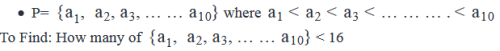Step 3: Analyze Statement 1 independently

(1) The difference between any two integers of the sequence is divisible by 2 and 3.

• Tells us that the difference between any two integers is of the form (2*3)k = 6k, where k is an integer > 0.

Does not tell us anything about the values of the integers of sequence P.

Step 4: Analyze Statement 2 independently

(2) If the third term of the sequence P is removed, the magnitude of the product of the terms of the sequence remains unchanged.

• So, the third term i.e. a3 = 1 or -1

The statement does not tell us anything about the other terms.

Step 5: Analyze Both Statements Together (if needed)

(1) Difference between any two integers is of the form (2*3)k = 6k

(2) a3 = 1 or -1

Combining both the statements, we cannot say for sure how many terms are less than 16, as we do know the value of 6k.

So, 6k = 6, 12 or 18.

For each case, we will have a different answer.

QUESTION: 5

Steven and Stuart took a job in different companies at the same time. Steven’s salary increased by a fixed amount at the end of every year and Stuart’s salary increased by a fixed percentage at the end of every year. If the increase in the salary of Steven at the end of the third year was equal to the increase in the salary of Stuart at the end of the second year, what was the difference in the salaries of Steven and Stuart when they took the job?

(1) Steven’s salary after 2 years was 20% more than the salary at which he took the job

(2) The increase in the salary of Stuart at the end of the second year was 11% of the salary at which he took the job.

Solution:

Steps 1 & 2: Understand Question and Draw Inferences

• Let Steven’s initial salary be S1

and Stuart’s initial salary be S1

•
• Let Steven’s salary increase by x every year and Steven’s salary increase by y% every year.
• Steven’s salary increase in the 3rd year = x
• Stuart’s salary increase at the end of the 2nd year  = y% of (Stuart’s salary in the 2nd year)
•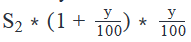• Steven’s salary increase in the 3rd year = Stuart’s salary increase in the 2nd year
•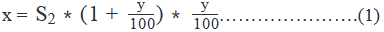To Find: S1−S2

Step 3: Analyze Statement 1 independently

(1) Steven’s salary after 2 years was 20% more than the salary at which he took the job

• Steven’s salary after 2 years =S1+2x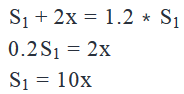However, we do not know anything about the values of S2, x and y.

Step 4: Analyze Statement 2 independently

(2) The increase in the salary of Stuart at the end of the second year was 11% of the salary at which he took the job.

• Increase in Stuart’s salary at the end of the 2nd year =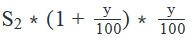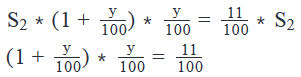Insufficient to answer as it does give us the value of  S1,S2orx

Step 5: Analyze Both Statements Together (if needed)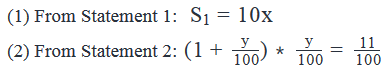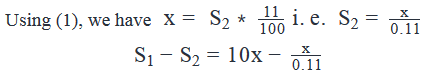Need to know the value of x to answer the question.

QUESTION: 6

If the sum of the first five terms of an Arithmetic sequence is equal to 120 and the sum of the next five terms of the same Arithmetic Sequence is equal to 245, what is the 4th term of this Sequence?

Solution:

Given:

• Sum of the first 5 terms of an arithmetic sequence = 120
• Sum of the next 5 terms of the same arithmetic sequence = 245
• Let the first term of this arithmetic sequence be x1 and let the common difference be d.

To Find:

• 4th term of the arithmetic sequence.
• So the 4th term of the sequence will become x1+3d
• So we need to find the value of x1 and d or the value of x1+3d to find the 4th term of the sequence.

Approach:

• We know that the sum of first n terms of the Arithmetic Sequence is given as
•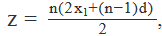where n is the number of terms in the arithmetic sequence.
• Using the formula above for the sum of first 5 terms of the sequence, we will get an equation in terms of  and common difference d, as we are given the sum of first 5 terms of the sequence.
• We are also given the sum of next 5 terms of the sequence. So, we will be able to calculate the sum of first 10 terms of the sequence.
→ Sum of first 10 terms of sequence = Sum of first 5 terms + sum of next 5 terms.
• Using the formula above for the sum of first 10 terms of sequence, we will get another equation in terms of x1 and common difference d.
• Using these two equations in x1 and d, we will be able to calculate the value of x1 and d.
• Knowing the values of x1 and d, we will be able to calculate the fourth term of the sequence, which is equal to x1+3d

Working out:

• Sum of first 5 terms of the arithmetic sequence = 120
• Putting this in formula of sum of first n terms, where n=5 and z=120, we get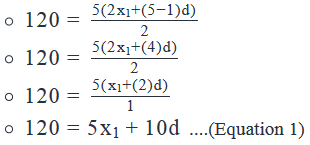• Sum of the next 5 terms of the sequence = 245

• Sum of the first 10 terms of the sequence = Sum of the first five terms + Sum of the next five terms.
• Sum of the first 10 terms of the sequence = 120+245 = 365

• Now, using the formula of the sum of first n terms of an arithmetic sequence, we get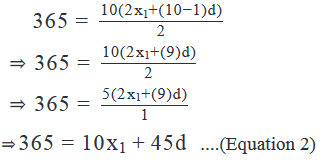• Solving Equations 1 and 2.
• Multiplying ‘equation 1’ by 2, we have 10x1+20d  =240 ...(Equation 3)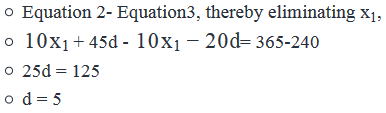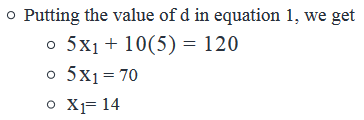Now that we have values of x1  and d. The value of 4th term of the sequence will be

⇒ x1+3(d)=14+3(5)=29

• The value of 4th term of the sequence is 29.
• Hence the correct answer is option A

Alternate method

• Let the first term be 'a' and common dfference between any two cosecutive terms be 'd'

Therefore,

• 1st term = a
• 5th term = a + 4d
• 6th term = a + 5d
• 10th term = a + 9d
• Average of first five terms of an arithemetic sequence  = (First term + Last term)/2 = (a + a +4d) / 2 = a + 2d
• Sum of first five terms = Average of first five terms * 5 = (a + 2d) * 5 = 120
• a + 2d = 120/5 = 24 ---------------- Eq(1)
• Average of next five terms of the arithemetic sequence  = (First term + Last term)/2 = (a+ 5d + a +9d) / 2 = a + 7d
• Sum of five terms = Average of five terms * 5 = (a + 7d) * 5 = 245
• a + 7d = 245/5 = 49---------------- Eq(2)

Solving Eq(1) and (2) we get

• d = 5
• 4th term =
• a + 3d = (a+2d)+ d = 24 + 5 = 29

QUESTION: 7

A list contains distinct integers a1, a2, …a10 arranged in ascending order. If the integers of the list lie between -19 and 19, inclusive such that the distance between any two consecutive integers is equal, is one of the terms of this list equal to zero?

(1) All the integers in the list are divisible by 2

(2) a4 = -6

Solution:

Steps 1 & 2: Understand Question and Draw Inferences

• a1, a2, …a10  is an arithmetic sequence
• Maximum Range of the sequence = 19 –(-19)= 38
• If a1 = -19, the maximum value of common difference can be for a value where a10 ≤ 19
• a1+ 9d ≤ 19, d ≤ 4.2
• Possible values of d = {1, 2, 3, 4}

Step 3: Analyze Statement 1 independently

(1) All the integers in the list are divisible by 2

• As all the terms of the sequence are even, the difference between any two consecutive terms should also be even.
• So, possible values of d can be {2 or 4}
• d =2, the range of the sequence will be 9d = 18. Let’s try to see if we can form a sequence that contains all the positive or negative terms
• If a1 = -18, a10 = a1 +9d=  -18 +9*2 = 0
• If a10 = 18, a1 = a10 – 9d = 18 – 9*2 = 0
• For all the other sequences consisting of both positive and negative terms, 0 will be a part of the sequence

• d= 4, the range of the sequence will be 9d = 36
• If a1 = -18, a10 = a1 +9d = -18 +36 = 18. The terms of the sequence = {-18,-14,-10,-6,-2,2…18}. Hence, 0 is not a part of the sequence.
• There is no other sequence possible with d = 4

As we do not have a unique answer, the statement is insufficient to answer the question

Step 4: Analyze Statement 2 independently

(2) a4 = -6

• Difference between -6 and 0 = 6
• 0 will be a part of the sequence if d = {1,2 or 3}
• If d= 4, 0 will not be a part of the sequence

Hence, cannot say for sure if 0 is a part of the sequence. Insufficient to answer

Step 5: Analyze Both Statements Together (if needed)

(1) d = {2, 4}

(2) a4 = -6

• If d = 2, 0 will be a part of the sequence
• If d= 4, 0 will not be a part of the sequence.

QUESTION: 8

An increasing sequence consists of 4 negative integers and 6 positive integers. Is the sum of the sequence positive?

(1) The difference between any two consecutive negative integers is 5 and the difference between any two consecutive positive integers is 2

(2) The first term of the sequence is -16

Solution:

Steps 1 & 2: Understand Question and Draw Inferences

• Let the sequence be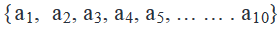where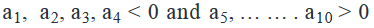To Find: Is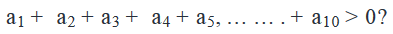Step 3: Analyze Statement 1 independently

(1) The difference between any two consecutive negative integers is 5 and the difference between any two consecutive positive integers is 2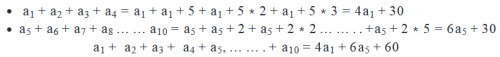Need to know the value of aand a to know if the sum of the sequence is greater than 0.

Step 4: Analyze Statement 2 independently

(2) The first term of the sequence is -16

• a1=−16
• We do not know the difference between consecutive terms

Hence,

• Statement 2 alone is not sufficient

Step 5: Analyze Both Statements Together (if needed)

From Statement 1 we got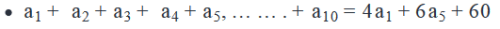From Statement 2 we got

• a1 = -16

Combining both we get: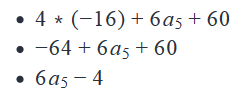Now, if we notice carefully a5   is a positive integer,

• a5 ≥ 1

Therefore

• 6a5≥6

Hence

• 6a5−4 is always greater than zero.

Combining both statements was sufficient to answer the question

QUESTION: 9

An increasing sequence M consists of 5 consecutive positive multiples of a positive integer. What is the remainder when the largest term of the sequence is divided by 2?

(1) The median of the sequence is even.

(2) The second term of the sequence is odd.

Solution:

Steps 1 & 2: Understand Question and Draw Inferences

• Let the positive integer of which the multiples are present in sequence M be p
• Let the terms of the sequence be {p*d, p*(d+1)……….p*(d+4)}

To Find:

• Remainder when the largest term of the sequence is divided by 2
• That is remainder when p*(d+4)  is divided by 2
• Any positive integer divided by 2 leaves a remainder 0 or 1
• If p * (d +4) is even, then remainder will be 0
• If p* (d +4) is odd, then remainder will be 1
• r = { 0 or 1}.
• So we need to determine if p*(d+4) is even or odd.
• p*(d+4) will be odd, if both the terms 'p' and 'd'  are odd.
• p*(d+4) will be even, if atleast one of the term is even.

Step 3: Analyze Statement 1 independently

(1) The median of the sequence is even.

• The median of sequence = p*(d+2) is even. Following cases are possible:
• Only p is even. In this case, p*d(+4) will be even.
• Only (d+2) is even. In this case, d+4 will be even, hence p*(d+4) will be even
• Both p and (d+2) are even. In this case, p*(d+4) are even.

Alternate method

• If p*(d+2) is even, we can write
• p*(d+4) = p*(d+2+2) = p*(d+2) + 2p = even + even = even
• Thus, p*(d+4) will always be even.

Step 4: Analyze Statement 2 independently

(2) The second term of the sequence is odd.

• p*(d+1) is odd, which is possible only if:
• p and (d+1) both are odd.
• If (d+1) is odd, (d+4) will be even. Hence p*(d+4) will be even.

Step 5: Analyze Both Statements Together (if needed)

As we have a unique answer from steps 3 and 4, this step is not required.

QUESTION: 10

Mike took 5 mock tests before appearing for the GMAT. In each mock test he scored 10 points more than the previous mock test. If he scored 760 on the GMAT and his average score for the mocks was 720, what is the difference between his last mock score and his GMAT score?

Solution:

Given

Mike took 5 mock tests

• Let his score in the 1st mock be x.
• So, his scores in the other mocks = x+ 10, x+20, x+30, x + 40
• Mike’s score on GMAT = 760
• Average score of mocks = 720

To Find:

• 760 – (x +40)

Working Out

Average score of mocks = (x + x + 10 + … x + 40) /5 = (5x + 100)/5 = x + 20
(The other way to think about this is, as Mike’s scores in the mocks are in arithmetic sequence, average will be the middle term)

• Mike’s average score in the mocks = x + 20 = 720
• x = 700

Therefore

• x + 40 = 740
• 760 – (x+40) = 760 – 740 = 20

QUESTION: 11

In the sequence S, the difference between any two consecutive terms is equal. If the sum of the fourth term and the fifth term of the sequence is equal to the seventh term of the sequence, what is the value of the second term of the sequence?

Solution:

Given

• In a sequence S, difference between any two terms is equal
• S is an arithmetic sequence
• Let the first term of the sequence be a and the common difference be d
• 4th   term + 5th  term = 7th  term
• 4th  term = a + (4-1)*d = a+ 3d
• 5th  term = a + (5-1)*d = a +4d
• 7th term = a + (7-1)d = a + 6d

To Find: a + d = ?

Approach

1. To find the value of a + d, we need to either find the values of a and d or a + d
2. We are given a relation
1. (a + 3d) + (a + 4d) = a + 6d
2. As we are given only one equation between a and d, we will be unable to find the values of both a and b. So, we will try to see if we can find the value of a + d

Working Out

1. (a + 3d) + (a+4d) = a+ 6d
1. 2a + 7d = a + 6d
2. a + d = 0

Hence, the value of the 2nd term of the sequence = a + d = 0

QUESTION: 12

A city had 1000 migrants in the year 1999. If the number of migrants in the city has doubled every 3 years since 1999, then what was the increase in the population of migrants during the period from 2008 to 2011?

Solution:

Given

• Number of migrants in the year 1999 = 1000
• Number of migrants doubles every 3 years

To Find:

• Increase in migrants population from 2008 to 2011?
• Migrants Population in 2011 – Migrants Population in 2008

Approach

1. As we know that the migrants population doubles every 3 years and migrants population in 1999 = 1000, we can use this information to calculate the migrants population in the year 2008 and the year 2011.

Working Out

1. Migrants population in year 1999 = 1000
2. Migrants population after (2008 – 1999 = 9 years) i.e. 3 intervals of 3 years = 1000 * 23
3. Migrants population after (2011 – 1999 = 12 years) i.e. after 4 intervals of 3 years= 1000 * 24
4. Difference in migrants population = 1000 *(24−23)=1000∗8=8000
1.

Hence, the migrants population increased by 8000 between 2008 and 2011.

QUESTION: 13

An arithmetic sequence is a sequence in which each term after the first is equal to the sum of the preceding term and a constant, which is also known as the common difference of that arithmetic sequence. The sequence S contains all the terms of two different increasing arithmetic sequences P and Q such that the number of terms in sequence S is equal to the sum of the number of terms in sequences P and Q. If each of the arithmetic sequences P and Q consists of 10 positive integral terms, how many distinct terms does sequence S have?

(1) The least common multiple of the common differences of the sequences P and Q is 6

(2) The third term of the sequence P is equal to the second term of the sequence Q

Solution:

Steps 1 & 2: Understand Question and Draw Inferences

• Increasing arithmetic sequence P with 10 positive integral terms with a common difference of p
• Increasing arithmetic sequence Q with 10 positive integral terms with a common difference of q.
• Sequence S consists of all the terms of sequences P and Q only, i.e. it consists of 20 terms only

To Find: Number of distinct terms of sequence S

• Number of distinct terms in sequence S = 20 – number of terms that are common to sequences P and Q ( as sequence S consists of terms from sequences P and Q only)
• To find the number of terms that are common to sequences P and Q, we need to find:
• The smallest term that is common to both the sequences
• The common difference of both the sequences i.e. values of p and q.

Step 3: Analyze Statement 1 independently

(1) The least common multiple of the common differences of the sequences P and Q is 6

• We are given the LCM of the common differences of the sequences P and Q is 6.
• 6 = 2 * 3.
• Hence the common differences (p, q) can be (1, 6) , (2, 6), (3, 6), (6, 6) or (2, 3) in any order
• So, we not have the unique values of the common difference of the two sequences
• Also, we do not know the smallest term, which is common to both the sequences.

Step 4: Analyze Statement 2 independently

(2) The third term of the sequence P is equal to the second term of the sequence Q

• We are given that P3 = Q2
• We do not know if these are the smallest  terms of the sequences P and Q that are common.Let us assume that these are not the smallest terms that are common to sequences P and Q. So, the possible cases can be: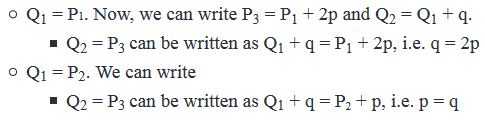• All the three cases are possible(i.e. Q1 and P1, or Q1 and P2 or Q2 and P3 are the smallest terms of the sequences that are common). So we cannot identify the smallest terms of the sequences P and Q that are common.

Also, we do not know the common difference of the two sequences. Hence the statement is insufficient to answer.

Step 5: Analyze Both Statements Together (if needed)

1. Common differences of sequences P and Q = (1, 6) , (2, 6), (3, 6), (6, 6) or (2, 3) in any order
2. P3 = Q2

Uisng (1) and (2), we cannot calculate the unique common values of the common differences as well as we do not know if P3 and Q2 are the smallest terms of the sequences P and Q that are common.

QUESTION: 14

An arithmetic sequence is a sequence in which each term after the first is equal to the sum of the preceding term and a constant, which is also known as the common difference of that arithmetic sequence. An increasing arithmetic sequence N consists of a set of distinct negative integers and an increasing arithmetic sequence P consists of a set of distinct positive integers. The sequence C contains all the terms of arithmetic sequences N and P such that the number of terms in sequence C is equal to the number of terms in arithmetic sequences N and P. Is sequence C an arithmetic sequence?

(1) The sum of the largest term of the sequence N and the smallest term of the sequence P is zero.

(2) For every integer in sequence N, there exists an integer in sequence P with the same magnitude.

Solution:

Steps 1 & 2: Understand Question and Draw Inferences

• Increasing arithmetic sequence N consist of distinct negative integers with common difference n
• Increasing arithmetic sequence P consist of distinct positive integers with common difference m.
• Sequence C consist of terms of sequences N and P only.

To Find: Is sequence C an arithmetic sequence?

• As sequence C consists of terms of arithmetic sequences N and P only, for sequence C to be arithmetic, sequences N and P should have the same common difference.

• Also, sequences N and P will not have any common terms (as sequence N has all negative integers and sequence P has only positive integers), for sequence C to be arithmetic, the difference between the largest term of sequence N and the smallest term of sequence P should be equal to the common difference of the sequences.

• For ex: Consider N = { -10, -9, -8……-1) and P = { 2, 3, 4, 5, ….}. In this case, both the sequences N and P have the same common difference but combining their terms does not result in an arithmetic sequence because the difference between -1 and 2 is not equal to the common difference of the sequences.

• Consider N = { -14, -10, -6, -2} and P = {2, 6, 10}. In this case, as the difference between -2 and 2 is equal to the common differences of the sequences(i.e. 4), combining the terms of both the sequences results in an arithemetic sequence.

Step 3: Analyze Statement 1 independently

(1) The sum of the largest term of the sequence N and the smallest term of the sequence P is zero.

• Let the largest term of sequence N be x and the smallest term of the sequence P be y.
• So, x + y = 0
• x = -y
• So, difference between y and x = y – x = 2y

However we do not know the value of the common differences of the sequences as well as we do not know the value of y.

Step 4: Analyze Statement 2 independently

(2) For every integer in sequence N, there exists an integer in sequence P with the same magnitude.

• It tells us that integers in sequence P consist of negative of all the integers in sequence N. Following cases are possible:
• Number of terms of sequence N and P are the same. For ex: N= {-8, -6, -4} and P = {4, 6, 8}. In this case the common difference of both the sequences will be equal.
• Number of terms in sequence P is greater than the number of terms in sequence N. For ex: N = {-8, -6, -4} and P = {3, 4, 5, 6, 7, 8, 9} or { 4, 6, 8, 10, 12}. In this case the common difference may or may not be equal.

Also, as we do not know the difference between the largest term of sequence N and the smallest term of sequence P, the statement is insufficient to answer.

Step 5: Analyze Both Statements Together (if needed)

1. Difference between the largest term of sequence N( i.e. x) and the smallest term of sequence P(i.e. y) is equal to 2y.
2. For every integer in sequence N, there exists an integer in sequence P with the same magnitude

Combining both the statements tell us that the largest term of sequence N (i.e. x) and the smallest term of sequence P(i.e. y ) have the same magnitude.

However it does not tell us:

• the value of y and
• if the sequences have the same common difference

Hence, combining the statements is also insufficient to answer.

QUESTION: 15

The sequence S consists of 10 terms: x, x2, x3……x10, where x is a non-zero number. If P is the sum of all the terms in the sequence S, is P3

> 0?

(1) The distance of any term of the sequence S from zero on the number line is not less than 1.

(2) x5 = x7

Solution:

Steps 1 & 2: Understand Question and Draw Inferences

• Sequence S= {x,x2,x3,…….x10
• P = x+x2+x3+…….+x10

To Find: Is P3> 0 ?

• P3 > 0. If P > 0 (Since P is raised to an odd power that does not affects its sign)
• As P = x+x2+x3……….+x10 , the positive or negative nature of P will depend on the positive or negative nature of x. Hence, following cases can arise:
• If x > 0 → In this case, x,x2,x3,…….x10 > 0. So, their sum will also be positive. Hence P > 0
• If x < 0 → The terms with an odd power i.e. x,x2,x3,…….x9 < 0 and the terms with an even power i.e. x,x2,x3,…….x10 > 0. So, the value of P will depend on the sum of the terms with an odd power and the terms with an even power. Also we know that if -1 < x < 0, with increasing power the magnitude of the terms decrease ( |x| > |x2|, while if x < -1, the magnitude of the terms increase with increasing power(|x| < |x2|). So, the following cases are possible:

•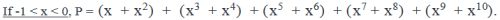• To understand the nature of P, let’s evaluate the first term of P in the equation above i.e.x + x2. Here x < 0 and x2 > 0. Since the magnitude of x is less than 1, the magnitude of x2 will be less than x. For ex: if x = -0.5, x2 = 0.25. Hence x + x2 < 0. Similarly x3 + x4 < 0. So, we see here that P is the sum of 5 negative numbers. Hence P < 0.
•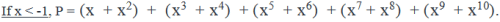• To understand the nature of P, let’s evaluate the first term of P in the equation above i.e. x + x2. Here x < -1 and x2 > 0. Since the magnitude of x is greater than 1, the magnitude of x2 will be greater than x. For ex: if x = -2, x2 = 4. Hence x + x2 > 0. Similarly x3 + x4 > 0. So, we see here that P is the sum of 5 positive numbers. Hence P > 0.
• However there may be  a case where the terms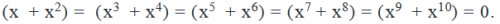• This would be possible when x(x+1) = 0, i.e. x = 0 or -1. As x≠ 0, for x = -1, P = 0.

So, P > 0, except when -1 < x < 0. Hence, we need to find if -1 < x < 0.

Step 3: Analyze Statement 1 independently

(1) The distance of any term of the sequence S from zero on the number line is not less than 1.

• As the distance of all the terms of the sequence from zero is not less than 1, the distance of the first term i.e. |x| ≥ 1. If |x| ≥ 1, ∣∣x2∣∣≥1………………….∣∣x10∣∣≥1
• So, x ≥ 1 or x ≤ -1. Following cases can arise: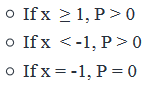So, we cannot say for sure if P > 0 or not. Insufficient to answer.

Step 4: Analyze Statement 2 independently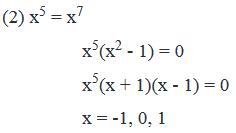• If x = -1, P = 0
• x ≠ 0(given in the question statement)
• If x = 1, P > 0

So, we cannot say for sure if P > 0. Insufficient to answer

Step 5: Analyze Both Statements Together (if needed)

1. x ≥ 1 or ≤ -1
2. x = -1 or 1

Combining both the statements, we have x = -1 or 1.

• If x = -1, P = 0
• If x = 1, P > 0

So, we cannot say for sure if P > 0.

QUESTION: 16

A sequence S consists of 5 distinct positive integers. Are all the integers in the sequence divisible by 5?

(1) The sum of all the integers in the sequence is divisible by 5.

(2) The product of all the integers in the sequence is divisible by 5 but not by 10.

Solution:

Steps 1 & 2: Understand Question and Draw Inferences

• Sequence S consists of a1,a2,a3,a4,a5
• , where all the terms are integers > 0

To Find: Is a1,a2,a3,a4,a divisible by 5?

Step 3: Analyze Statement 1 independently

(1) The sum of all the integers in the sequence is divisible by 5.

a1+a2+a3+a4+a5=5x

, where x is an integer > 0.

Two cases arise:

• All of a1,a2,a3,a4,a5 are a multiple of 5. In this case all the terms are divisible by 5
• Two or more terms sum up to a multiple of 5. In this case all the terms are not divisible by 5.

Step 4: Analyze Statement 2 independently

(2) The product of all the integers in the sequence is divisible by 5 but not by 10.

a1∗a2∗a3∗a4∗a5=5y, where y is an integer > 0

For the product of the terms to be divisible by 5, any one or more of the terms should be divisible by 5.

So, 1 or all the 5 terms may be divisible by 5.

Also, as the product of the terms is not divisible by 10, none of the terms is even.

Step 5: Analyze Both Statements Together (if needed)

(1) a1+a2+a3+a4+a5=5x

(2) a1∗a2∗a3∗a4∗a5=5y  and none of a1,a2,a3,a4,a is even

Following cases may arise:

• All the terms are odd multiples of 5.
• 1 or more terms are an odd multiple of 5 and the rest terms are odd and not a multiple of 5 but sum up to be a multiple of 5.

QUESTION: 17

The sequence a1, a2,…an is such that an = an-1 +n*d for all n > 1, where d is a positive integer. If a3 = 20 and a5 = 47, what is the value of a7?

Solution:

Given

• A sequence a1, a2,…an
• an = an-1 +n*d for all n > 1, where d is an integer > 0
• a3 = 20
• a5 = 47

To Find: a7?

Approach

1. As an = an-1 +n*d, we can express a7 in terms of a1 and d
1. So, we need to find the value of a1 and d.
2. As we are given the values of a3 and a5, we will express them in terms of a1 and d to get 2 equations in a1 and d.
3. We will then solve these two equations to find out the value of a1 and d.

Working Out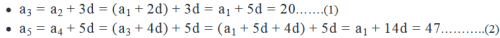Solving (1) and (2), we have a1 = 5 and d = 3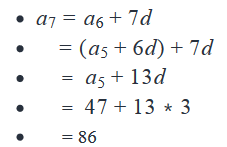QUESTION: 18

For any positive integer z, SZ denotes the sum of the first z positive integers. For example S3 = 1+2 + 3 = 6. Which of the following expressions is correct?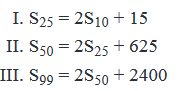Solution:

Given:

• For a positive integer z, Sz = 1 + 2 + 3 + .  .  . + z

To find: Which of the given 3 expressions is correct?

Approach:

1. Since the 3 expressions are independent of one another (that is, they do not talk about the same value or item), we will evaluate the expressions one by one, using the given definition of SZ

Working Out: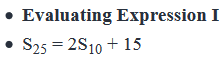• Now, by definition, S25 is the sum of the first 25 integers.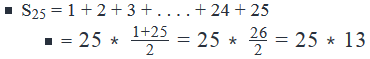By definition,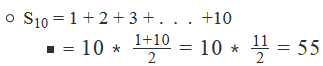• So, 2S10 + 15 = 2*55 + 15
• This value is clearly much smaller in magnitude than 25*13
• Therefore, Expression 1 is not true.

• Evaluating Expression II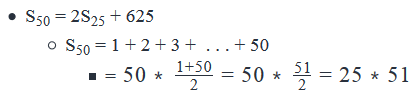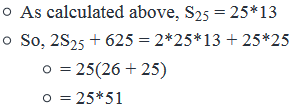• Thus, we see that S50 is indeed equal to 2S25 + 625
• Therefore, Expression II is correct
• Evaluating Expression III
• S99 = 2S50 + 2400
• Note: You can calculate Expression III using the formula for the sum of an arithmetic sequence. The working out for this method will be similar to the working out shown above for Expressions I and II. Alternatively, you can solve as below. This is an alternate way of evaluating the given expressions. This way can also be used to evaluate Expressions I and II.
• S99 = 1 + 2 + 3 + . . . . + 99
• = (1 + 2 + 3 + . . . + 99 + 100) – 100
• = (1 + 2 + 3 + . . . + 50) + (51 + 52 + . . . + 100) - 100
• = S50 + {(50+1) + (50 + 2) . . . + (50 + 50)} – 100
• = S50 + 50*50 + (1 + 2 + 3 + . . . + 50) – 100
• = S50 + 2400 + S50
• = 2S50 + 2400
• Thus, Expression III is indeed correct
• Conclusion
• So, we have determined that of the 3 given expressions, only Expressions II and III are correct.

Looking at the answer choices, we see that the correct answer is Option E

QUESTION: 19

Ted and Robin start from the same point at 7 AM and drive in opposite directions. Ted doubles his speed after every 90 minutes whereas Robin reduces her speed by half after every 120 minutes. If Ted starts driving at a speed of 10 kilometers/hour and Robin starts driving at a speed of 120 kilometers/hour, how far in kilometers will they be from one another at 1 PM?

Solution:

Given

• Ted starts at 7 AM
• Ted’s initial speed = 10 kilometers/hour
• Ted doubles his speed after every 1.5 hours

• Robin starts at 7 AM from the same point as Ted and travels in opposite directions
• Robin’s initial speed = 120 kilometers/hour
• Robin reduces her speed by half after every 2 hours

To Find: Distance between Ted and Robin at 1 PM

• Find the distance travelled by Ted in 6 hours
• Find the distance travelled by Robin in 6 hours

Approach

Distance = Speed * Time

1. We need to find the distance travelled by Ted in 6 hours As Ted doubles his speed after every 1.5 hours, he will travel at 6/1.5=4

1.  different speeds during the period
2. We know his initial speed and we know that he doubles his speed after every interval of 1.5 hours. So, we can calculate his speed for each interval

2.  We need to find the distance travelled by Robin in 6 hours

1. As Robin reduces her speed after every 2 hours, she will travel at 6/2=3
1.  different speeds during the period
2. We know her initial speed and we know that she reduces her speed by half after every interval of 2 hours. So, we can calculate her speed for each interval.

Working Out

1.  Ted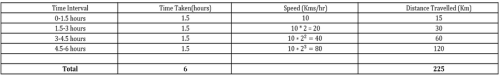2.  Robin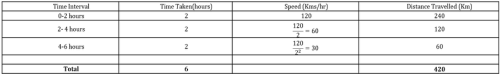Distance between Ted and Robin at 1 PM = 225 + 420 = 645 kilometers

QUESTION: 20

List A consists of 10 distinct integers arranged in ascending order. Is the difference between the sixth term and the fifth term of list A greater than 5?

(1) The difference between any two integers in list A is a multiple of 5.

(2) The median of the list is an integer.

Solution:

Given:

1. List A = {a1, a2, a3, a4, a5, a6, a7, a8, a9, a10}
1. consists of all integers,
2. where a10 > a9> a8…> a2> a1

To Find:  Is a6 – a5 > 5?

Step 4: Analyse Statement 2 independently

The median of the list is an integer.

• Median =?
• List contains 10 elements
• a5 & a6 are integers at the centre of the list (in ascending order)
• Median of the list = a5+a62
•  = m, where m is an integer
• Rearranging, we get a5+ a6 = 2m = even
• If sum of a6 & a5 is even, then
• Difference of a6 & a5 is also even
• No information about values of the terms , so cannot tell if (a6 – a5) > 5
• Hence, statement 2 is insufficient to answer the question.

Step 5: Analyse Both Statements Together (if needed)

• From statement 1, a6 – a5 = {5, 10, 15}
• From statement 2, a6 – a5 = even
• Combining the two,
• a6 – a5 = even multiples of 5 = {10, 20, 30…}
• a6 – a5 > 5
• So, we can answer the question.
• Hence statement 1 and statement 2 together are sufficient to arrive at a definite answer.Use Code STAYHOME200 and get INR 200 additional OFF Use Coupon Code